Next: Heisenberg's Uncertainty Principle Up: Wave-Particle Duality Previous: Wave Packets

# Evolution of Wave Packets

We have seen, in Eq. (86), how to write the wavefunction of a particle which is initially localized in-space. But, how does this wavefunction evolve in time? Well, according to Eq. (83), we have(97)

where(98)

The function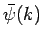is obtained by Fourier transforming the wavefunction at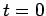. See Eqs. (89) and (93). Now, according to Eq. (95),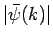is strongly peaked around. Thus, it is a reasonable approximation to Taylor expand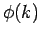about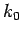. Keeping terms up to second-order in, we obtain(99)

where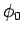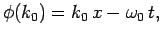(100)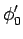(101)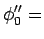(102)

with(103)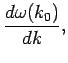(104)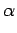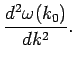(105)

Substituting from Eq. (93), rearranging, and then changing the variable of integration to, we get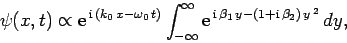(106)

where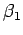(107)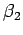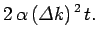(108)

Incidentally,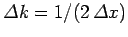, where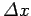is the initial width of the wave packet. The above expression can be rearranged to give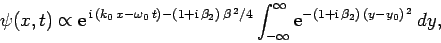(109)

where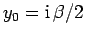and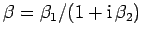. Again changing the variable of integration to, we get(110)

The integral now just reduces to a number. Hence, we obtain(111)

where(112)

Note that the above wavefunction is identical to our original wavefunction (86) at. This, justifies the approximation which we made earlier by Taylor expanding the phase factorabout.

According to Eq. (111), the probability density of our particle as a function of time is written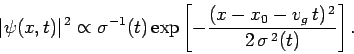(113)

Hence, the probability distribution is a Gaussian, of characteristic width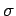, which peaks at. Now, the most likely position of our particle coincides with the peak of the distribution function. Thus, the particle's most likely position is given by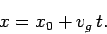(114)

It can be seen that the particle effectively moves at the uniform velocity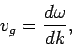(115)

which is known as the group velocity. In other words, a plane wave travels at the phase velocity,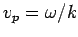, whereas a wave packet travels at the group velocity,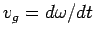. Now, it follows from the dispersion relation (79) for particle waves that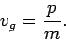(116)

However, it can be seen from Eq. (71) that this is identical to the classical particle velocity. Hence, the dispersion relation (79) turns out to be consistent with classical physics, after all, as soon as we realize that individual particles must be identified with wave packets rather than plane waves. In fact, a plane wave is usually interpreted as a continuous stream of particles propagating in the same direction as the wave.

According to Eq. (112), the width of our wave packet grows as time progresses. Indeed, it follows from Eqs. (79) and (105) that the characteristic time for a wave packet of original widthto double in spatial extent is(117)

For instance, if an electron is originally localized in a region of atomic scale (i.e.,) then the doubling time is only about. Evidently, particle wave packets (for freely moving particles) spread very rapidly.

Note, from the previous analysis, that the rate of spreading of a wave packet is ultimately governed by the second derivative of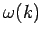with respect to. See Eqs. (105) and (112). This is why a functional relationship betweenandis generally known as a dispersion relation: i.e., because it governs how wave packets disperse as time progresses. However, for the special case whereis a linear function of, the second derivative ofwith respect tois zero, and, hence, there is no dispersion of wave packets: i.e., wave packets propagate without changing shape. Now, the dispersion relation (50) for light waves is linear in. It follows that light pulses propagate through a vacuum without spreading. Another property of linear dispersion relations is that the phase velocity,, and the group velocity,, are identical. Thus, both plane light waves and light pulses propagate through a vacuum at the characteristic speed. Of course, the dispersion relation (79) for particle waves is not linear in. Hence, particle plane waves and particle wave packets propagate at different velocities, and particle wave packets also gradually disperse as time progresses.Next: Heisenberg's Uncertainty Principle Up: Wave-Particle Duality Previous: Wave Packets
Richard Fitzpatrick 2010-07-20